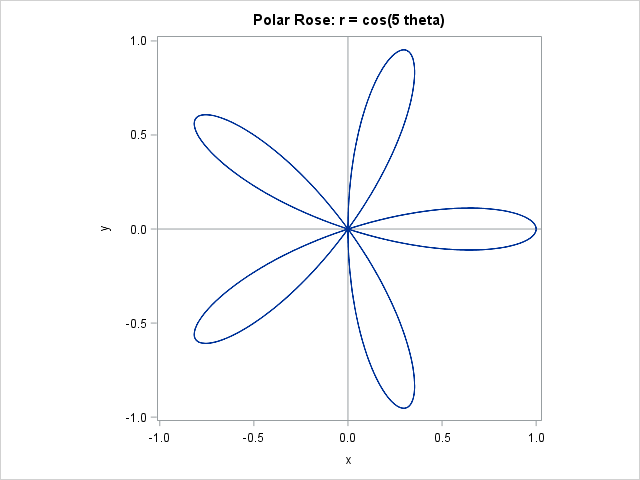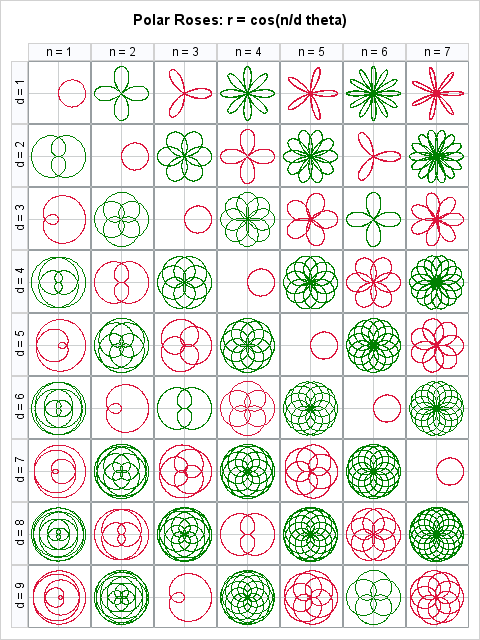Lo how a rose e'er blooming
From tender stem hath sprung.

As I write this blog post, a radio station is playing Chrismas music. One of my favorite Christmas songs is the old German hymn that many of us know as "Lo, How a Rose E're Blooming." I was humming the song recently when someone asked me how to use SAS to draw curves in polar coordinates. I immediately thought about "polar roses," mathematical parametric curves of the form r = cos(k θ).

In the spirit of Christmas, I present "Lo, how a polar rose e'er blooming, from SGPLOT hath sprung."

### Plotting polar coordinates in SAS

It is easy to graph a polar curve in SAS. Here's a quick overview, or a "polar express" tour, if you prefer.

Many familiar polar equations are parametric curves. The simplest are relations are of the form r = f(θ), where θ is the polar angle. The following SAS DATA step creates evenly spaced values of theta in the range [0, 2π]. For each value of theta, it computes r = cos(k θ), which is the equation for a rose with k petals. The polar coordinates (r, θ) are converted to Euclidean coordinates through the usual transformation x = r*cos(theta) and y = r*sin(theta).

After creating the points along the curve, you can visualize it by using PROC SGPLOT. The SERIES statement is used to display the parametric curve in Euclidean coordinates. The XAXIS and YAXIS statements use the MIN= and MAX= options to ensure that the image appears in a square region, and the ASPECT=1 option is used to ensure that the aspect ratio of the plot does not distort the geometry of the rose.

```%let k = 5; /* draw the curve r=cos(k * theta) in polar coords, which is a k-leaved rose */ data Rose; do theta = 0 to 2*constant("pi") by 0.01; r = cos(&k * theta); /* rose */ x = r*cos(theta); /* convert to Euclidean coords */ y = r*sin(theta); output; end; run;   title "Polar Rose: r = cos(&k theta)";; proc sgplot data=Rose aspect=1; refline 0 / axis=x; refline 0 / axis=y; series x=x y=y; xaxis min=-1 max=1; yaxis min=-1 max=1; run;```### Graphing generalized roses

The Wikipedia page for the polar rose contains a brief discussion about what the curve r = cos(k θ) looks like when k is a rational number n/d. When k is not an integer, most of the curves do not look like a rose—or any other kind of flower! Only a myopic mathematician would call some of these images roses.

Regardless, let's see how to get some of these "roses" to bloom in SAS. The following DATA step generates all positive rational numbers of the form n/d, where n ≤ 7 and d ≤ 9 are integers. For each value of k, the program computes the generalize rose equation and converts the values from polar to Euclidean coordinates:

```data Roses; do n = 1 to 7; do d = 1 to 9; k = n / d; /* draw the rose r=cos(n/d * theta) */ do theta = 0 to 2*lcm(n,d)*constant("pi") by 0.05; r = cos(k * theta); /* generalized rose */ x = r*cos(theta); /* convert to Euclidean coords */ y = r*sin(theta); output; end; end; end; run;```

It is not any harder to visualize 63 roses than it is to visualize one. The SGPANEL procedures was designed to display multiple cells, each with the same type of graph. Therefore, to display an entire bouquet of roses, you merely have to specify the N and D variables on the PANELBY statement, and SAS handles the rest:

```title "Polar Roses: r = cos(n/d theta)"; proc sgpanel data=Roses aspect=1; panelby n d / layout=lattice onepanel rowheaderpos=left; refline 0 / axis=x transparency=0.5; refline 0 / axis=y transparency=0.5; series x=x y=y; colaxis display=none; rowaxis display=none; run;```You can see certain numeric patterns in the lattice display. In particular, when n/d is a reducible fraction (such as 2/4, 3/6, and 4/8), the image is identical to the reduced fraction (such as 1/2). Do you see other patterns? Leave a comment.

These roses are beautiful, like the song that inspired me to create them. Since I get up early in the morning to write my blog, a few additional lyrics seem appropriate:

It came a blossom bright.
Amid the cold of winter
When half spent was the night.

Happy holidays to all my readers. I am taking a short vacation and will return in 2016. Until then, keep your eyes open: math, statistics, and beauty are all around you, ever blooming!

tags: Just for Fun, Math

The post Lo, how a polar rose e'er blooming appeared first on The DO Loop.